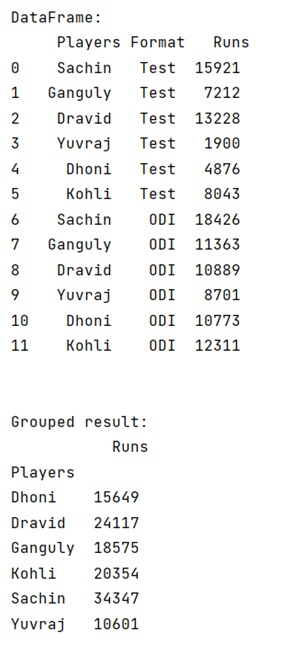# How to perform pandas groupby() and sum()?

Learn how to perform pandas groupby() and sum()? By Pranit Sharma Last updated : September 19, 2023

Pandas is a special tool that allows us to perform complex manipulations of data effectively and efficiently. Inside pandas, we mostly deal with a dataset in the form of DataFrame. DataFrames are 2-dimensional data structures in pandas. DataFrames consist of rows, columns, and the data. DataFrame can be created with the help of python dictionaries or lists but in the real world, CSV files are imported and then converted into DataFrames.

## Performing pandas groupby() and sum()

The groupby() is a simple but very useful concept in pandas. By using groupby(), we can create a grouping of certain values and perform some operations on those values.

The groupby() function split the object, apply some operations, and then combines them to create a group, hence large amount of data and computations can be performed on these groups.

One of the simplest methods on groupby objects is the sum() method.

Syntax:

```DataFrame.groupby(
by=None,
axis=0,
level=None,
as_index=True,
sort=True,
group_keys=True,
squeeze=NoDefault.no_default,
observed=False,
dropna=True
)
```

Let us understand with the help of an example:

## Python program to perform pandas groupby() and sum()

```# Importing pandas package
import pandas as pd

# creating a dictionary of cricketers
d = {
"Players":['Sachin','Ganguly','Dravid','Yuvraj','Dhoni','Kohli',
'Sachin','Ganguly','Dravid','Yuvraj','Dhoni','Kohli'],
"Format":['Test','Test','Test','Test','Test','Test',
'ODI','ODI','ODI','ODI','ODI','ODI'],
"Runs":[15921,7212,13228,1900,4876,8043,
18426,11363,10889,8701,10773,12311]
}

# Now we will create DataFrame
df = pd.DataFrame(d)

# Viewing the DataFrame
print("DataFrame:\n",df,"\n\n")

# Performing sum on groupby on Players with runs
result = df.groupby(['Players']).sum()

# Display Result
print("Grouped result:\n",result)
```

### Output

The output of the above program is: